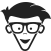You searched for - Page 9 of 8161 - dummies

Showing 96 Search Results for Joseph schmuller

There are no results for available. Check the spelling and try again, or check the most popular results from this topic.

Search Result
Sort By

96 results found.

•In Excel

October 20,2016

### How to Visualize a t-Distribution in Excel

Visualizing a distribution often helps you understand it. It’s easy with Excel, and it’s instructive. The image below shows how to do it for a t-d...

•In Excel

October 20,2016

### Using Worksheet Functions for Statistical Analysis

Excel 2016b has a variety of functions for statistical analysis. The Function Library area of the Formulas tab shows all categories of Excel worksheet...

•In Excel

October 20,2016

### How to Use the KURT Function in Excel

To calculate kurtosis in Excel, you use the KURT function. The first has a peak at its center; the second is flat. The first is said to be leptokurtic...

•In Excel

October 18,2016

### What’s New in Excel 2016 for Statistical Analysi

Microsoft has made a few changes to Excel’s Ribbon (the tabbed band across the top), reflecting changes in Excel. The most obvious addition is the l...

•In Excel

March 27,2016

### Statistical Analysis with Excel For Dummies Cheat

Excel offers a wide range of statistical functions you can use to calculate a single value or an array of values in your Excel worksheets. The Excel A...

•In Excel

March 26,2016

### Excel Array Functions for Statistical Analysis

An array formula calculates a set of values rather than just one. Here are Excel’s statistical array functions. Each one returns an array of val...

•In Excel

March 26,2016

### Some Excel Worksheet Functions for Statistical Ana

Excel can help you make all sorts of calculations. Here’s a selection of Excel’s statistical worksheet functions. Each one returns a value...

•In Excel

March 26,2016

### Excel Data Analysis Tools

Excel’s Analysis ToolPak is a helpful add-in that provides an extensive set of statistical analysis tools. Here are some of the tools in the Too...